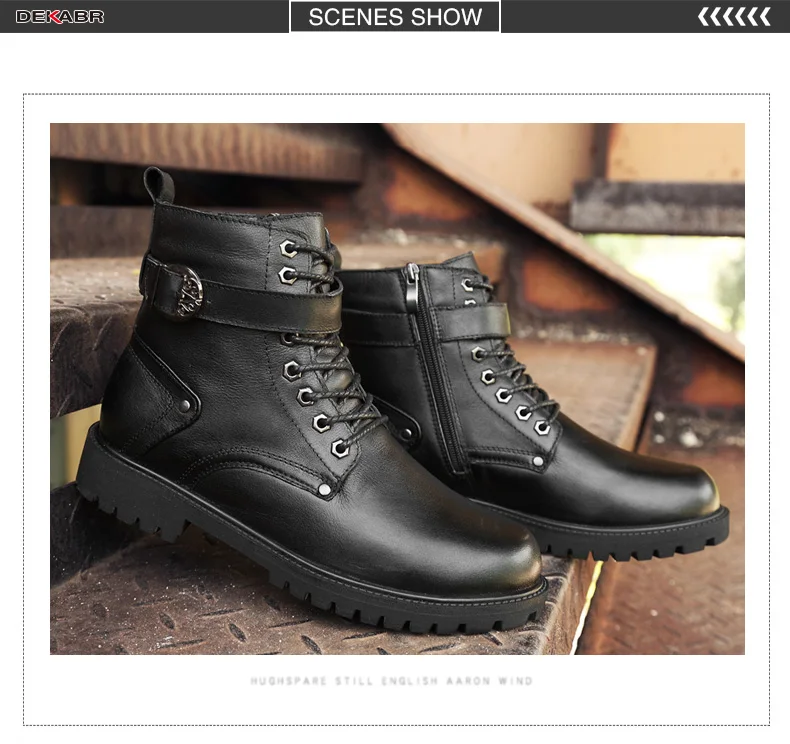\$119.99

# SPECIFICATIONS

Toe Shape: Round Toe

Style: British Style

Season: Winter

Pattern Type: Solid

Outsole Material: Rubber

Item Type: Boots

Comfortable: Ankle Boots

Color: Black

Closure Type: Lace-up

Size 5=35    fit for foot length is 23cm.

Size 5.5=36  fit for foot length is 23.5cm.

Size 6=37    fit for foot length is 24cm.

Size 6.5=38  fit for foot length is 24.5cm.

Size 7=39    fit for foot length is 25cm.

Size 7.5=40  fit for foot length is 25.5cm.

Size 8=41    fit for foot length is 26cm.

Size 8.5=42  fit for foot length is 26.5cm.

Size 9.5=43  fit for foot length is 27cm.

Size 10=44  fit for foot length is 27.5cm.

Size 11=45  fit for foot length is 28cm.

Size 12=46  fit for foot length is 28.5cm.